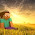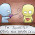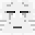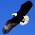## Tuesday, 5 March 2013

### Algebra... Different "Faces" of Algebra (I)

The total length of 4 roads is (3x - 1) km.
What are the possible lengths of the 4 roads in kilometres?

e.g. The length of the 4 roads are: x km, x km, x-7 km, 6 km
Check: x + x + (x - 7) + 6 = 3x - 1

1.This comment has been removed by the author.

1.This comment has been removed by the author.

2.x-1, x, 0.5x, 0.5x

1.Forgot the units. Its all in km

2.Correct but there are units missing (km).

3.(x+1)+(x+8)+(x-11)+1
Check x+1+x+8+x-11+1

1.2.Its correct. But presentation is for checking only. No conclusion

4.This comment has been removed by the author.

5.x-5 km, x+5 km, x-10 km, 9 km

1.6.x km, x/2 km, (x/2-1)km, x km

1.7.0.5x,1x,0.5x,1x + (-1)km
check 0.5x+0.5x+1x+(-1)
=(3x-1)km

1.0.5x+0.5x+1x+1x+(-1)

2.1X + (-1)km shld be (X-1)km

3.8.5,x+7,x-13,x

1.2.It's correct!

9.0.5x, 0.5x, x-1,x

1.This comment has been removed by the author.

2.You are right.

3.OH!
You forgot the (km) so you are wrong!

10.x km + x km + x+10 km+ x-9 km

1.Your answer is wrong Jian Qing. x km+x km is 2x km. 2x km + (x+10 km) is 3x +10 km. 3x + (x-9 km) is 4x + 1 km.

11.(X-10000, X-20000 , X+30000 , X-1)

1.3RD Value is 3000

2.I mean 30000

3.4.12.the lengths of the 4 roads are : x - 5km, x km, x + 1km, 3km

1.Correct-a-mungo!

13.(x-8)+x+x+7=3x-1

1.correct... but missed out "km" :|

2.and never state separate four lengths

14.This comment has been removed by the author.

15.x km + x km + 20km + x - 19km = 3 x -1

1.Correct. But x-19km should be in brackets and answer is missing the units.

16.x-1,2/3x,2/3x,2/3x

1.This comment has been removed by the author.

2.You're missing out on your units and the brackets for the fractions.

17.x+1, 0.5x, x-2, 0.5x

1.u are correct!B)

2.18.0.5x+0.5x+x+x+(-1)=(3x-1)

1.just write x-1...

2.but still correct

19.0.75x, 0.75x, 0.5x, x-1

1.all okay. Units? km/cm/m/\$/min?

20.0.5x KM, 0.5x KM, 0.5 x KM, 1.5 x KM -1

check: 0.5x +0.5x +0.5 + 1.5x-1 = 3x-1

1.2.*** 0.5x

u missed the x in 0.5, so is incorrect

21.x+x+100km+x-99

1.ITS CORRECT

2.But missing units

22.(x+1)+(x+7)+(x-10)+1 (km)

1.23.0.5x , 0.5x , 0.5x , 1.5x-1km

1.